### What are your chances of acceptance?Duke UniversityUCLA
Unweighted GPA: 3.7
1.0
4.0
SAT: 720 math
200
800
| 800 verbal
200
800

#### Extracurriculars

Low accuracy (4 of 18 factors)Thamira Skandakumar
11

# 10 Hardest AP Chemistry Exam Questions

### Do you know how to improve your profile for college applications?

See how your profile ranks among thousands of other students using CollegeVine. Calculate your chances at your dream schools and learn what areas you need to improve right now — it only takes 3 minutes and it's 100% free.

## What’s Covered:

In 2020, 56.1% of test takers passed the AP Chemistry exam with a 3 or higher. As you can see, the AP Chemistry exam is one of the more difficult exams administered by the College Board. But, with the right study tools, such as CollegeVine’s Ultimate Guide to the AP Chemistry Exam, and through lots of practice, you will be more than prepared to pass the exam!

In this article, we will review some of the more difficult questions you may encounter on the exam followed by detailed explanations describing how to solve them.

## How Will AP Scores Impact My College Chances?

The score you get on an AP exam, whether it be a 2 or a 5, won’t really impact your chances of admission into a particular college. Most colleges will not even review your scores, especially since you don’t have to send them in if you don’t want to!

College admissions will instead take a more holistic approach when reviewing your college application. Course rigor, GPA,  essays, and extracurriculars are more likely to be valued when reviewing your application. When it comes to course rigor, colleges want to see how many APs you take and what grades you get in the classes rather than the exam scores, which are used to determine course credit and placement after you get into college.

If you are interested in understanding how your course load impacts your chances at college, use our free chancing engine. This tool will help you determine your chances of acceptance at over 500 colleges based on your grades, test scores, extracurriculars and demographics. Keep in mind that your essays and letters of recommendation are also important in the college admissions process!## Overview of the AP Chemistry Exam

The AP Chemistry exam assesses your ability to understand science practices in relation to four big ideas: scale, proportion and quantity; structure and properties; transformations; and energy. The exam is 3 hours and 15 minutes long and is comprised of two separate sections:

Section I: Multiple Choice Questions

• 60 questions
• 90 minutes
• A calculator is permitted
• Worth 50% of your exam score

Section II: Free Response Questions(FRQs)

• 7 Questions
• 105 minutes
• A calculator is NOT permitted
• Worth 50% of your exam score

The AP Chemistry exam is broken up into nine units with the following percentage breakdowns:

• Atomic Structure and Properties (7-9%)
• Molecular and Ionic Compound Structure and Properties (7-9%)
• Intermolecular Forces and Properties (18-22%)
• Chemical Reactions (7-9%)
• Kinetics (7-9%)
• Thermodynamics (7-9%)
• Equilibrium (7-9%)
• Acids and Bases (11-15%)
• Applications of Thermodynamics (7-9%)

## 10 Hardest AP Chemistry Questions

In this article, we will be focusing on official CollegeBoard AP Chemistry Exam questions. This means that you can expect to see the same level of difficulty on the exam you take.

CollegeBoard is also known to provide answer options that are meant to confuse you into choosing the wrong answer instead of the correct one. But fear not! We will show you how to carefully read the question and make sure that you are confident in the answer you choose!

### Question 1In this first question, we see that a base Mg(OH)2 is being titrated by an acid HNO3. In order to determine the mass of the initial base, we need to perform a series of titration calculations. This is a great example of when your labs come in handy, since the lab can help you visualize what is going on.

First, we need to determine the reaction that is occurring when Mg(OH)2  is reacted with HNO3. We first write out the equation we know, which is that the base and acid react to create water and an aqueous solution of the remaining ions:

Mg(OH)2  + HNO3 → H2O + Mg2+ + NO3

We now need to balance the above reaction. Hydrogen is already balanced, so the next element we move to is Oxygen. We see that Oxygen is unbalanced with five oxygen on the left and four oxygen on the right. We can balance Oxygen by:

Mg(OH)2  + 2HNO3 → 2H2O + Mg2+ + 2NO3

This gives us 8 oxygen molecules on either side. This simple balancing act has now balanced the entire equation with one Mg molecule, 8 O molecules, 4 H molecules and 2 N molecules on either side.

Now we return to the titration problem. Since we know that the acid is being used as the titration solution, we know that HNO3 is in the buret. We also know that 20 mL is needed to get to the end point since:

22.17 mL – 2.17 mL = 20 mL

Now that we know the molar mass of the base (58.3 grams), the molarity of the acid solution, and the amount of acid needed to titrate the bass and the balanced equation, we can perform a simple stoichiometric equation. Make sure to balance all your units and note that it takes 2 moles of HNO3 for every one mole of Mg(OH)2!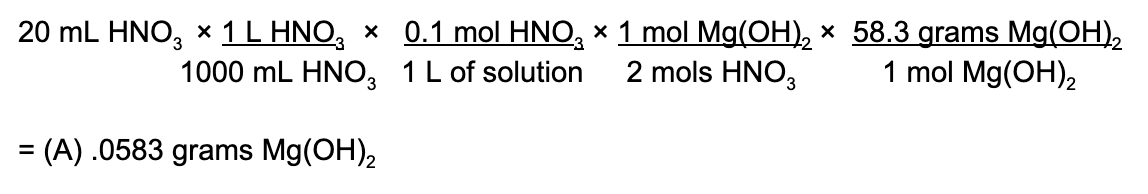### Question 2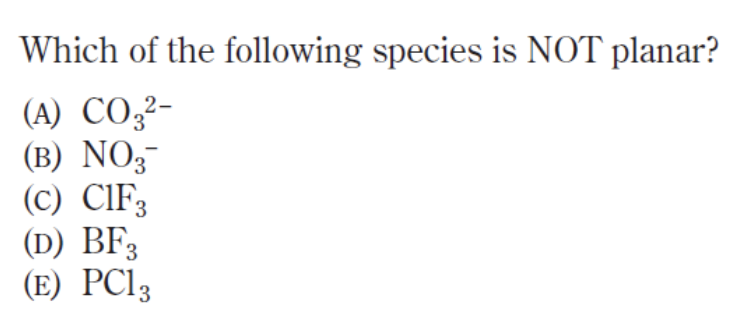Geometry of a molecule or compound is an important topic covered on the AP Chemistry exam so it is important that you know your tetrahedrals from your trigonal bipyramidials! An easy hint is that you can almost always determine what type of shape a compound is based on the number of lone electron pairs and molecules that are attached to the central atom.

That being said, a planar compound is one where the atoms surrounding the central molecule arrange themselves so that they exist on a singular, two-dimensional plane. In order to determine which of these is NOT planar (remember to read extra carefully!!!), we’ll draw out each of the molecules. Make sure to follow the quartet rule when drawing out each of the compounds!

CO32-:This carbonate ion, while experiencing negative charges on the oxygen, is planar since all oxygens are in the same plane as one another.

NO3: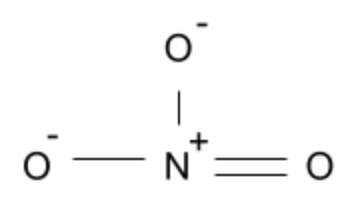The nitrate ion is also planar since the oxygen atoms are in the same plane as one another and are not impacted by lone pairs (similar to the Carbonate ion).

ClF3: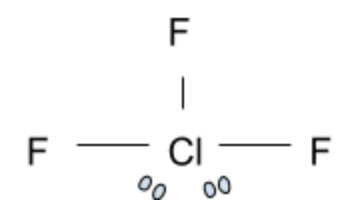Now this is a tricky compound. At first glance, you would assume that ClF3 is not planar due to the impact of the two electron pairs present. However, the chlorine trifluoride compound is actually a T-shaped geometrical structure. In this shape, the 3 Fluorine atoms are in the same plane and form a T shape with the Chlorine atom. Surprisingly, this is not the correct answer.

BF3: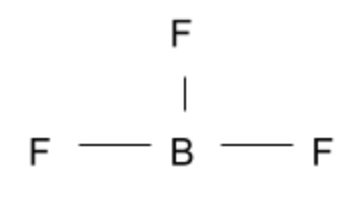This compound is another simple answer we can cross off since the three Fluorine atoms are in the same plane as one another.

PCl3:This leaves us with phosphorus trichloride. The electron pair causes a disruption in what would be expected of a planar compound. Each individual chlorine atom experiences great repulsion from the lone electron pair, thereby causing the Chlorine atoms to bend closer together. This gives this compound a trigonal pyramidal shape instead of a planar geometry.

Therefore, the compound that is not planar is (E) PCl3.

### Question 3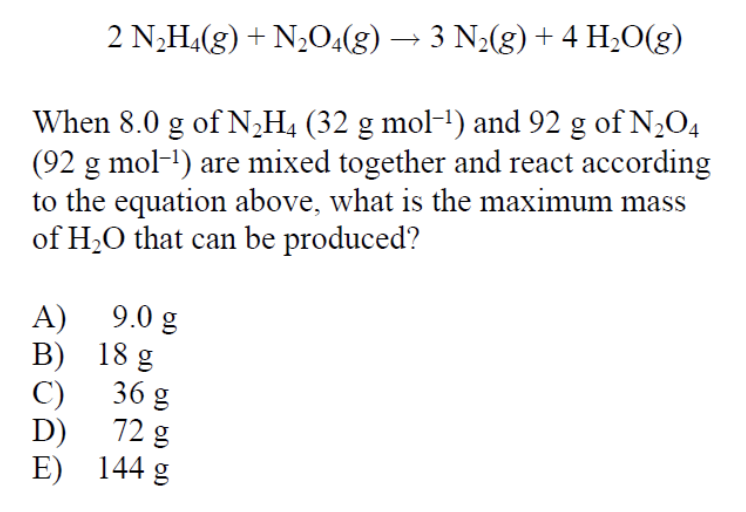This is a tricky question since we are given the initial mass of both reactants and are asked to find the maximum mass of a product, in this case water. This means this is a limiting reactant problem in which a limiting reactant limits how much a product can be produced.

Let’s perform stoichiometric equations on 8 grams of N2H4 as well as 92 grams of N2O4 to determine which of these reactants is the limiting reactant. But first, always, always, always double check that the provided equation is balanced! (Thankfully in our case, it is).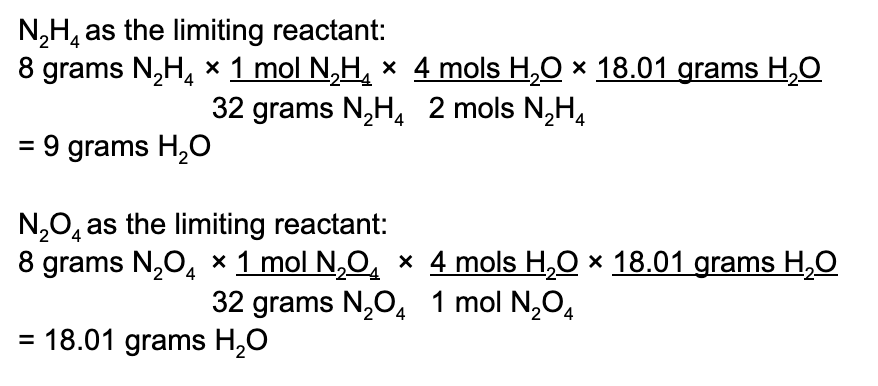Here we see that while N2O4 may produce the most amount of water, this is an inaccurate representation of what happens. N2H4 acts as a limiting reactant and limits how much water is produced. N2O4 is in excess. Therefore, the maximum amount of water produced is 9 grams.

## Discover your chances at hundreds of schools

Our free chancing engine takes into account your history, background, test scores, and extracurricular activities to show you your real chances of admission—and how to improve them.

### Question 4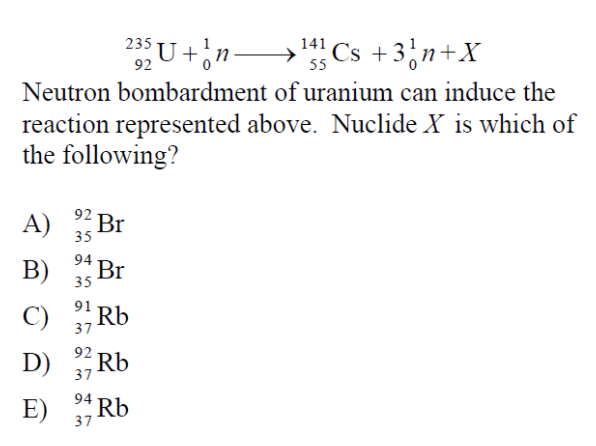This question is difficult since it’s not a problem that is often seen in class. The main thing we need to understand is that regardless of how difficult this question looks, ultimately, it’s another balancing problem!

In this reaction, neutrons are being used to induce a fission reaction. A neutron is the same mass as a proton, however, has no charge (no electrons or protons).

Remember that the upper number next to the element represents the atomic mass while the bottom number represents the atomic number.

To simplify the reaction we can expand the equation to account for the three neutrons that are being displaced:

92235U + 10n → 55141Cs +  01n + 01n + 01n + ??X

This means that we can perform simple subtraction to determine what ??X is since the law of conservation of mass must be obeyed:

(92)-(55)(235+1) – (141+1+1+1)X = 3792X

By checking a periodic table of elements, we see that Rb has an atomic mass of 92 and an atomic number of 37.

### Question 5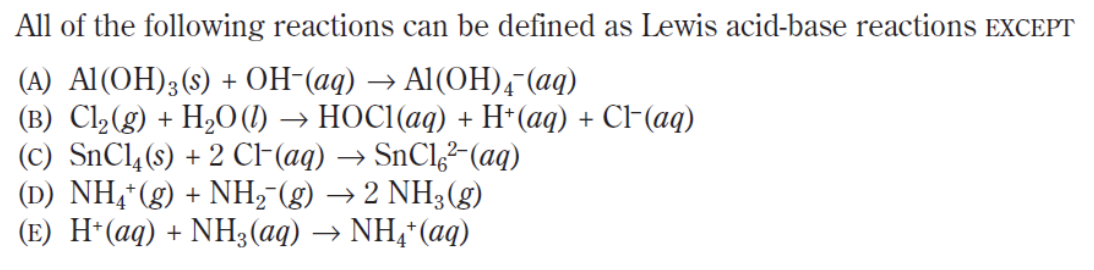This is another EXCEPT question so we have to be extra careful as to what is being asked of us! Remember that a Lewis acid-base reaction is one in which a Lewis-base donates it’s extra pair of electrons to a Lewis acid.

In the five options above, OH, CL, NH2 and NH3 have electron pairs that are capable of donating their additional electrons to the Lewis acid AL(OH)3, SnCl4, NH4+ and H+ respectively. In option (b), H2O acts as a nucleophile and binds with Cl2 and breaks the intramolecular Cl2 bond. While doing so, the additional hydrogen atom breaks off in order to stay true to the octet rule. In doing so, the reaction fails to create a complete covalent bond between the two molecules.

### Question 6This question is difficult because it requires you to understand how a buffer and titration works and know how to approximate data based on a graph.

The optimum buffer action occurs when the amounts of acid and its conjugate base are approximately equal. In the case of our situation, this happens when half of the weak acid has been neutralized to form its conjugate base.

It may seem like the best answer for this question is point W, the point when there is enough base to neutralize the acid (also known as the equivalence point). But this is incorrect! This point basically neutralizes the entire solution and that’s not what we’re looking for! At this point, the strong base is in excess in relation to the weak acid to guarantee that all of the weak acid is neutralized (as seen by the pH value of 8.0 at point W).

We are looking for the point when the buffer action is optimal, which as we defined before, is when there are equal amounts of acid and its conjugate base. This point occurs approximately midway between the equivalence point (at which the slope of the graph is nearly infinite) and the starting point. In relation to the graph this occurs at point V.

### Question 7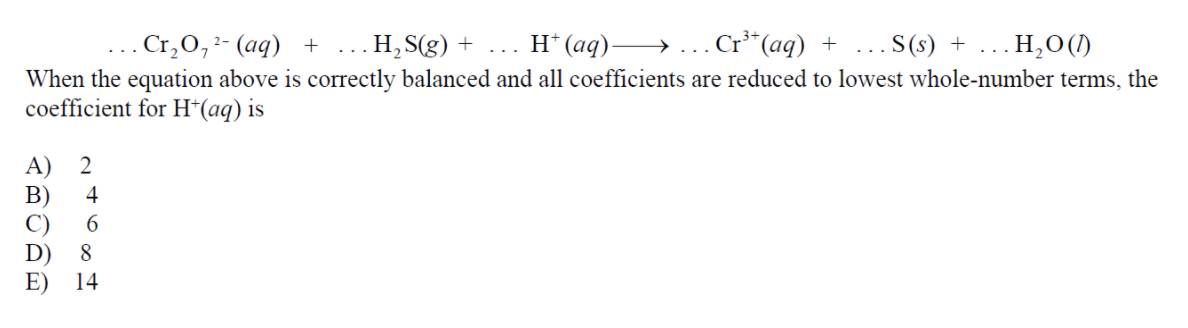Balancing equations always proves to be time consuming and difficult, especially when performed under pressure. However, it is almost guaranteed that these types of problems will appear on the exam.

This type of question is best solved as reduction and oxidation half reactions To do this, we need to determine which elements are being reduced and which elements are being oxidized. We see that the chromium ion gains electrons, therefore becoming reduced, while sulfur loses electrons when it becomes a solid, therefore becoming oxidized.

Reduction: Cr2O7(aq) + H+(aq) → Cr3+(aq) + H2O(l)

Oxidation: H2S(g) → S(s)

Next, we must balance all the atoms in the above equation. We can account for missing Hydrogen ions by adding in protons:

Reduction: Cr2O7(aq) + 14H+(aq) → 2Cr3+(aq) + 7H2O(l)

Oxidation: H2S(g) → S(s) + 2H+

From here, we must determine how many electrons are needed to balance out the charges of either side, which we can do by adding 6 electrons to the left side of the reduction equation and 2 electrons the the right side of the oxidation equation:

Reduction: Cr2O7(aq) + 14H+(aq) + 6e → Cr3+(aq) + 7H2O(l)

Oxidation: H2S(g) → S(s) + 2H+ + 2e

The next goal is to cancel out the electrons from either side. We can do this by multiplying the oxidation equation by 3. This then becomes:

Reduction: Cr2O7(aq) + 14H+(aq) + 6e → Cr3+(aq) + 7H2O(l)

Oxidation: 3H2S(g) → 3S(s) + 6H+ + 6e

Now we can add the respective equations to get:

Cr2O7(aq) + 14H+(aq) + 3H2S(g) +  6e  → Cr3+(aq) + 7H2O(l) + 3S(s) + 6H+ + 6e

The last step is to cancel out any like terms on opposite sides:

Cr2O7(aq) + 8H+(aq) + 3H2S(g)  → Cr3+(aq) + 7H2O(l) + 3S(s)

Therefore, the coefficient of H+ is 8!

### Question 8This question requires us to remember concepts of solubility constant as well as how reactions work in order to conserve mass. The first thing we must do is to write out the reaction that is described in the problem statement. The thing that makes this question difficult is writing out the correct reaction. Why you may ask? It’s because the correct equation involves balancing out the charges of the atoms!

Zn(OH)2 → 2OH + Zn2+

We know that Ksp is written as the product of the concentrations of the aqueous ions in a solution. Since OH has a coefficient of 2, we must square the concentration of the base. Therefore:

Ksp = [OH]2[Zn2+]

From here, we are dealing with easy math! We know that the hydroxide ion and Zinc need to dissolve in proportional concentrations. Therefore, we can reduce the equation in terms of x. But, don’t forget to include the coefficient of 2 in front of the hydroxide ion as well!

Ksp = [2x]2[x]

We are given the concentration of the hydroxide ion as 2.0 x 10-6 M. This means that:

2x = 2.0 x 10-6 M

x = 1.0 x 10-6 M

Plugging these values into the above equation gives us:

Ksp = [2.0  x 10-6]2[1.0 x 10-6] = 4.0 x 10-18 M

### Question 9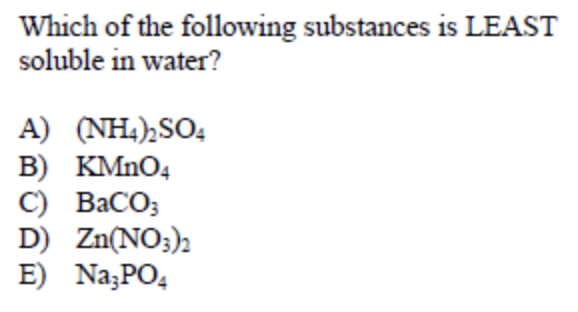This type of question seems difficult to look at since you are immediately presented with numerous compounds you may have not seen before. With this type of question, it is important to remember characteristics about specific compounds such as halides and sulfates.

One fact to remember about carbonates (CO32-) is that they are most often insoluble. They are especially insoluble when paired with Group II atoms such as Barium, Calcium and Strontium. Therefore, BaCO3 is the least soluble in water of the groupings.

### Question 10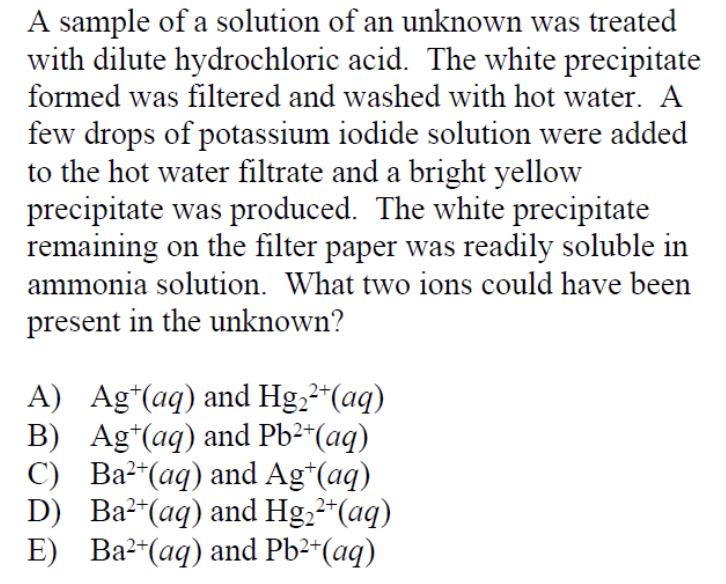This is another one of those questions where it is extremely helpful if you are able to remember labs shown or conducted in your class. This question also requires you to remember qualitative characteristics of compounds to deduce what ions could be present in an unknown solution.

The initial white precipitate formed corresponds to a chloride precipitate since hydrochloric acid was used to treat the unknown solution. However, we know that BaCl2 is soluble, so we can deduce that Barium is not in the initial solution since a solid forms after the treatment.

This leaves us with AgCl, HgCl2 and PbCl2 as potential precipaties. Since a bright yellow precipitate formed after the introduction of the potassium iodide solution, we know that this is a characteristic of lead iodide. Therefore, lead is present in the solution. This leaves us with answer choice (B) as the right answer.

To verify that B is correct, we must determine that silver is also present in the unknown solution. We know this to be true because silver easily dissolves in ammonia, as described in the problem statement.

## Final Tips

As you prepare for this exam, remember to:

Many of the multiple choice questions and free response questions you’ll come across will describe lab scenarios. These scenarios may have been ones you have previously seen in class or in a video. It’ll be easier for you to understand and correctly answer the question at hand if you are able to visualize what is being described based on something you have seen previously in class!

### Practice, Practice, Practice!

You may know the concepts very well, but you also need to be able to apply the concepts in a timed setting. Make sure you take multiple practice tests administered through CollegeBoard or through your school. You might be able to answer every question on the test perfectly, but you need to be sure that you can finish the multiple choice section in 90 minutes! Remember, that leaves just about one and a half minutes to read the question and determine the answer. Over time, you will get better at quickly answering questions and determining what the question is asking for on your first try!

### The Night Before…

Review your important equations! Know what each equation on the formula sheet means. It’s one thing to know what the variables on the equation sheet mean, but it’s another to know why you would use an equation and how it can help you solve any question at hand.

People will always tell you to get a good eight hours of sleep and eat a healthy breakfast. While this may seem like basic info that is not relevant to the test material you are memorizing, it is important that you are awake and ready to sit through a three hour exam!Short Bio
My name is Thamira Skandakumar and I am from Vancouver, Washington. I am a current fourth year at UCLA studying Bioengineering with a minor in Electrical Engineering. In the summer, I will be moving out to San Diego to work at Medtronic in their Ventilator division.